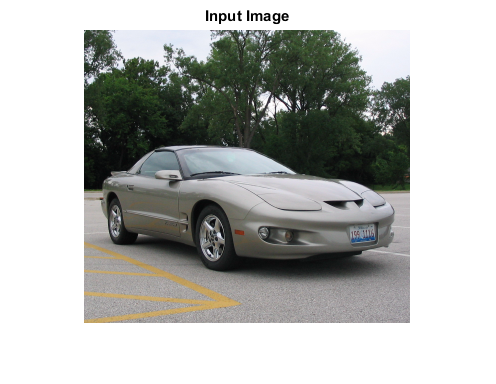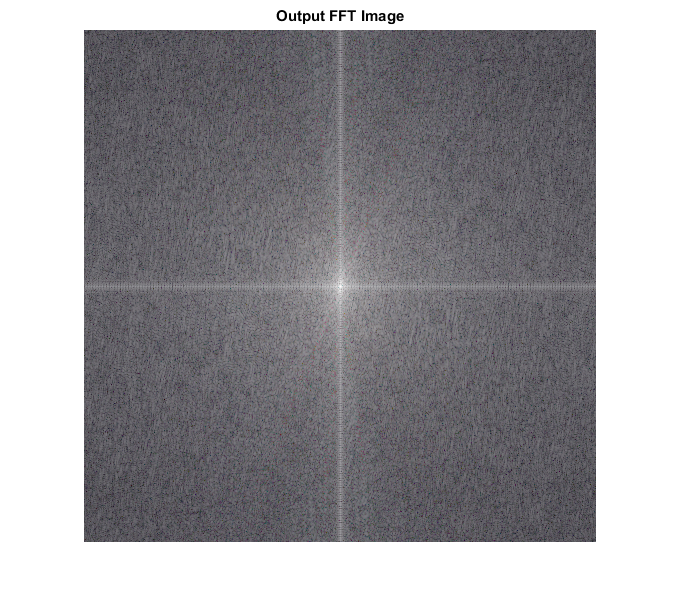# FFT_CVIP

fft_cvip() - performs Fast Fourier Transform.

## SYNTAX

`spect = fft_cvip( input_img, block_size )`

Input parameters include:

• input_img - The original image which can be grayscale or RGB.
• block_size - Should be a power of 2 or [ ]. if empty, then functions finds the nearest power of 2 and zero pad the image, to that block size. Any other value smaller than image size will partiotion the image to windows of that block size and FFT will be calculated separately in each window.

Output parameters include:

• spect - Resultant spectrum of the image after FFT.

## DESCRIPTION

This function computes the fourier transform of the input image. The argument block_size is usually given empty brackets [] so that the function handles the zero padding itself. Any other value for block_size results in dividing the area of the image into squares of size block_size and taking the fourier of the small windows.

## REFERENCE

`1. Scott E Umbaugh. DIGITAL IMAGE PROCESSING AND ANALYSIS: Applications with MATLAB and CVIPtools, 3rd Edition.`

## EXAMPLE

``` input_img = imread('Car.bmp');

% Block size

block_size = [];

% Call function

spect = fft_cvip( input_img, block_size );

% use abs to compute the magnitude (handling imaginary) and use log to brighten display

S2=log(1+abs(spect));

S2 = remap_cvip(S2);

% Display input image

figure; imshow(input_img);title('Input Image');

% Display output image

figure; imshow(S2,[]);title('Output FFT Image');
```